# Newtonmass fractals 2018

It is Newtonmass, and that means doing some fun math. I try to invent a new fractal or something similar every year: this is the result for 2018.

The Newton fractal is an old classic. Newton’s method for root finding iterates an initial guess$z_0$ to find a better approximation$z_{n+1}=z_{n}-f(z_{n})/f'(z_{n})$. This will work as long as$f'(z)\neq 0$, but which root one converges to can be sensitively dependent on initial conditions. Plotting which root a given initial value ends up with gives the Newton fractal.

The Newton-Gauss method is a method for minimizing the total squared residuals$S(\beta)=\sum_{i=1}^m r_i^2(\beta)$ when some function dependent on n-dimensional parameters $\beta$ is fitted to$m$ data points$r_i(\beta)=f(x_i;\beta)-y_i$. Just like the root finding method it iterates towards a minimum of$S(\beta)$:$\beta_{n+1} = \beta_n - (J^t J)^{-1}J^t r(\beta)$ where$J$ is the Jacobian$J_{ij}=\frac{\partial r_i}{\partial \beta_j}$. This is essentially Newton’s method but in a multi-dimensional form.

So we can make fractals by trying to fit (say) a function consisting of the sum of two Gaussians with different means (but fixed variances) to a random set of points. So we can set$f(x;\beta_1,\beta_2)=(1/\sqrt{2\pi})[e^{-(x-\beta_1)^2/2}+(1/4)e^{-(x-\beta_1)^2/8}]$ (one Gaussian with variance 1 and one with 1/4 – the reason for this is not to make the diagram boringly symmetric as for the same variance case). Plotting the location of the final$\beta(50)$ (by stereographically mapping it onto a unit sphere in (r,g,b) space) gives a nice fractal:Basins of attraction of different attractor states of Gauss-Newton’s method. Two Gaussian functions fitted to five random points.Basins of attraction of different attractor states of Gauss-Newton’s method. Two Gaussian functions fitted to 10 random points.Basins of attraction of different attractor states of Gauss-Newton’s method. Two Gaussian functions fitted to 50 random points.

It is a bit modernistic-looking. As I argued in 2016, this is because the generic local Jacobian of the dynamics doesn’t have much rotation.

As more and more points are added the attractor landscape becomes simpler, since it is hard for the Gaussians to “stick” to some particular clump of points and the gradients become steeper.

This fractal can obviously be generalized to more dimensions by using more parameters for the Gaussians, or more Gaussians etc.

The fractality is guaranteed by the generic property of systems with several attractors that points at the border of two basins of attraction will tend to find their ways to other attractors than the two equally balanced neighbors. Hence a cut transversally across the border will find a Cantor-set of true basin boundary points (corresponding to points that eventually get mapped to a singular Jacobian in the iteration formula, like the boundary of the Newton fractal is marked by points mapped to$f'(z_n)=0$ for some n) with different basins alternating.

Merry Newtonmass!

# Newtonmas fractals: conquering the second dimension!

It is Newtonmas, so time to invent some new fractals.

Complex iteration of Newton’s method for finding zeros of a function is a well-known way of getting lovely filigree Julia sets: the basins of attraction of the different zeros have fractal borders.

But what if we looked at real functions? If we use a single function$f(x,y)$ the zeros will typically form a curve in the plane. In order to get discrete zeros we typically need to have two functions to produce a zero set. We can think of it as a map from R2 to R2$F(x)=[f_1(x_1,x_2), f_2(x_1,x_2)]$ where the x’es are 2D vectors. In this case Newton’s method turns into solving the linear equation system$J(x_n)(x_{n+1}-x_n)=-F(x_n)$ where$J(x_n)$ is the Jacobian matrix ($J_{ij}=dF_i/dx_j$) and$x_n$ now denotes the n’th iterate.

The simplest case of nontrivial dynamics of the method is for third degree polynomials, and we want the x- and y-directions to interact a bit, so a first try is$F=[x^3-x-y, y^3-x-y]$. Below is a plot of the first and second components (red and green), as well as a blue plane for zero values. The zeros of the function are the three points where red, green and blue meet.

We have three zeros, one at$x=y=-\sqrt{2}$, one at$x=y=0$, and one at$x=y=\sqrt{2}.$ The middle one has a region of troublesomely similar function values – the red and green surfaces are tangent there.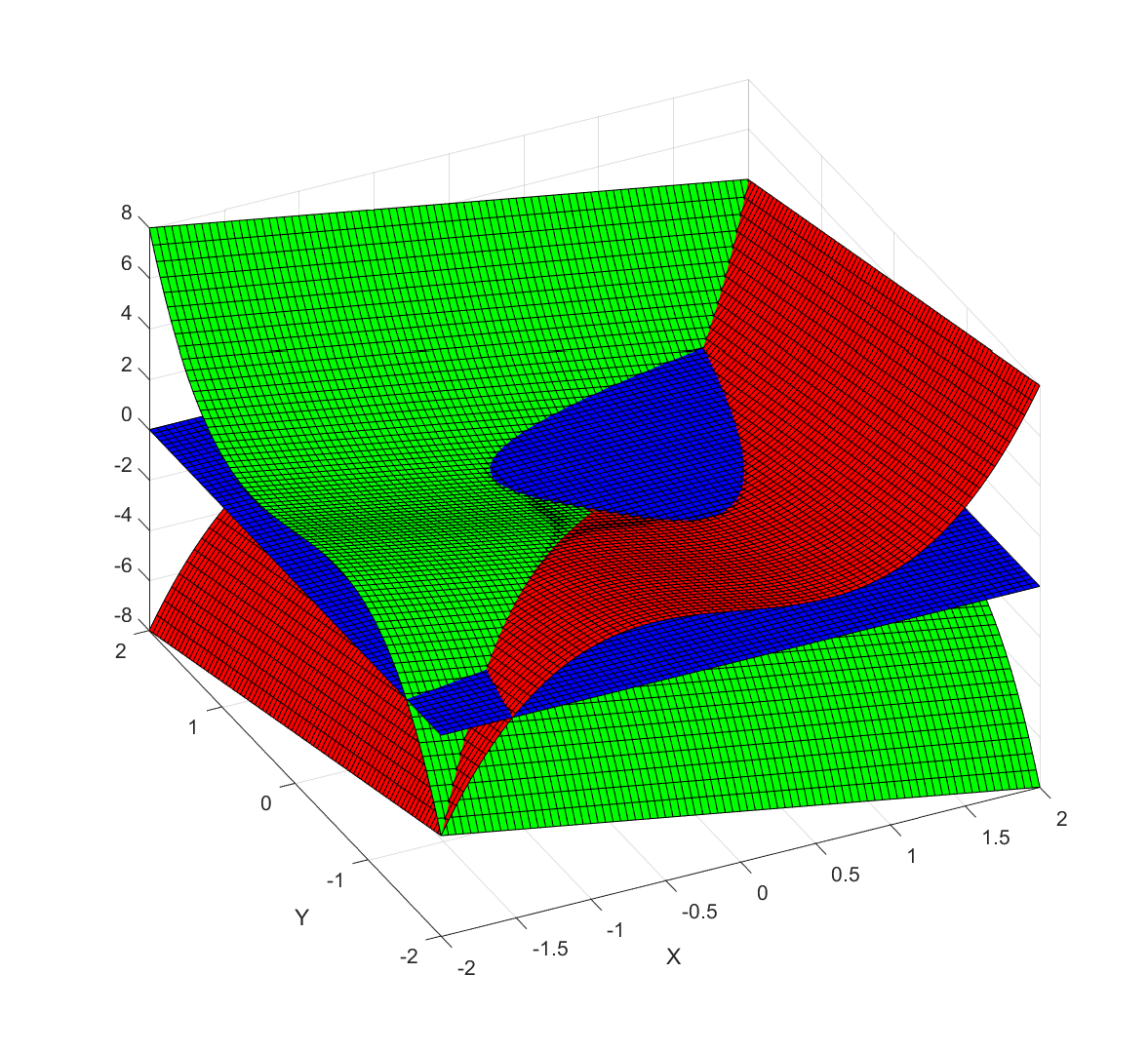Plot of x^3-x-y (red), y^3-x-y (green) and z=0 (blue). The zeros found using Newton’s method are the points where red, green and blue meet.

The resulting fractal has a decided modernist bent to it, all hyperbolae and swooshes: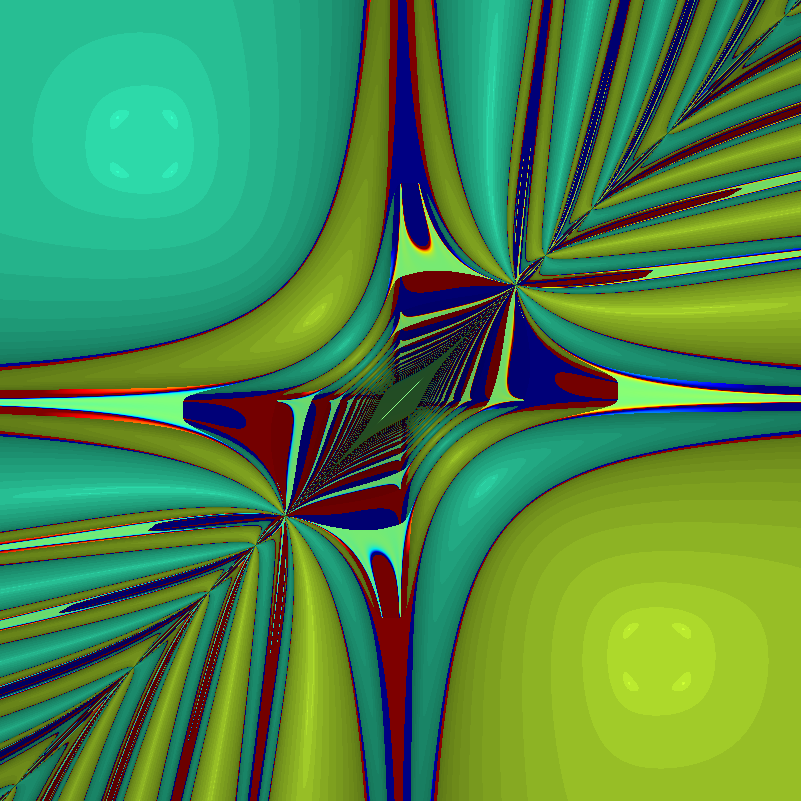Behavior of Newton’s method in 2D for F=[x^3-x-y, y^3-x-y]. Color denotes value of x+y, with darkening for slow convergence.The troublesome region shows up, as well as the red and blue regions where iterates run off to large or small values: the three roots are green shades.

# Why is the style modernist?

In complex iterations you typically multiply with complex numbers, and if they have an imaginary component (they better have, to be complex!) that introduces a rotation or twist. Hence baroque filaments are ubiquitous, and you get the typical complex “style”.

But here we are essentially multiplying with a real matrix. For a real 2×2 matrix to be a rotation matrix it has to have a pair of imaginary eigenvalues, and for it to at least twist things around the trace needs to be small enough compared to the determinant so that there are complex eigenvalues:$T^2/4 (where$T=a+d$ and$D=ad-bc$ if the matrix has the usual$[a b; c d]$ form). So if the trace and determinant are randomly chosen, we should expect a majority of cases to be non-rotational.

Moreover, in this particular case, the Jacobian tends to be diagonally dominant (quadratic terms on the diagonal) and that makes the inverse diagonally dominant too: the trace will be big, and hence the chance of seeing rotation goes down even more. The two “knots” where a lot of basins of attraction come together are the points where the trace does vanish, but since the Jacobian is also symmetric there will not be any rotation anyway. Double guarantee.

Can we make a twisty real Newton fractal? If we start with a vanilla rotation matrix and try to find a function that produces it the simplest case is$F=[x \cos(\theta) + y \sin(\theta), x\sin(\theta)+y\cos(\theta)]$. This is of course just a rotation by the angle theta, and it does not have very interesting zeros.

To get something fractal we need more zeros, and a few zeros in the derivatives too (why? because they cause iterates to be thrown away far from where they were, ensuring a complicated structure of the basin boundaries). One attempt is$F=[ (x^3-x-y) \cos(\theta)$$-(y^3-x-y) \sin(\theta),$$(x^3-x-y) \sin(\theta)+(y^3-y-x) \cos(\theta) ]$. The result is fun, but still far from baroque: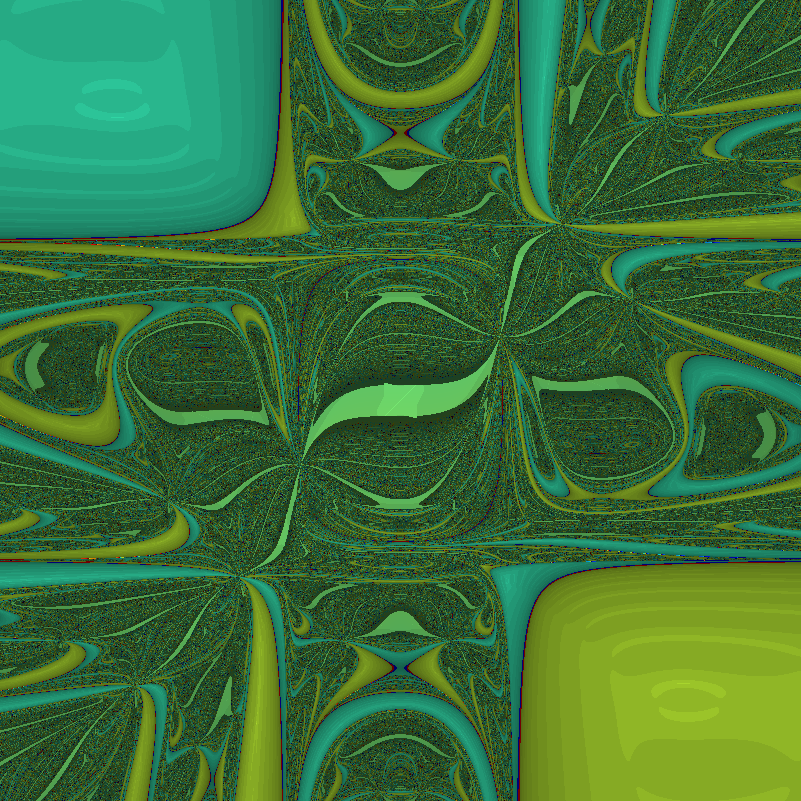Basins of attraction for Netwon’s method of twisted cubic. theta=1.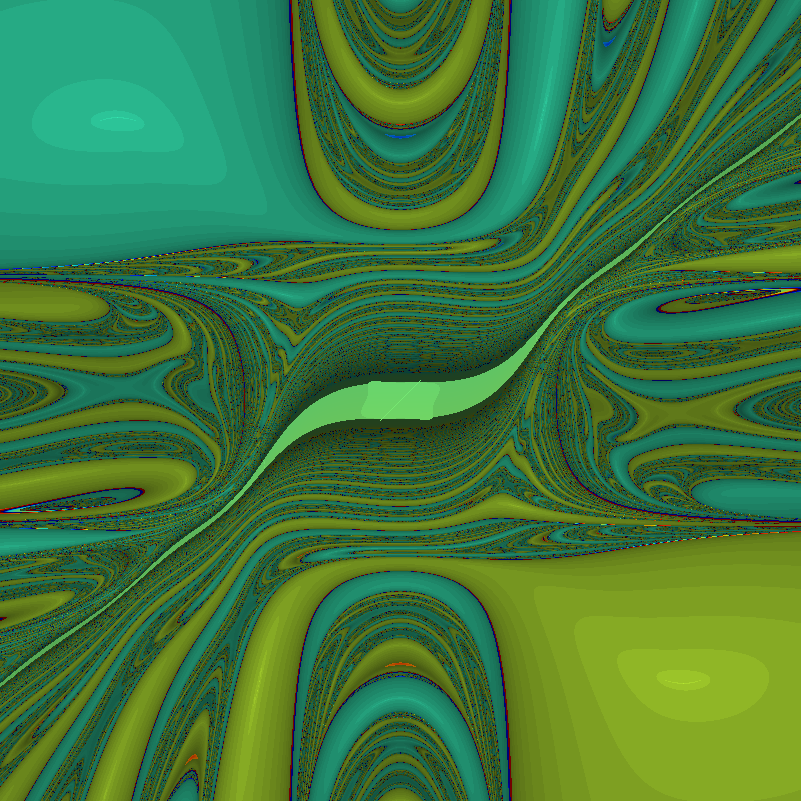Basins of attraction for Netwon’s method of twisted cubic. theta=0.1.

The problem might be that the twistiness is not changing. So we can make$\theta=x$ to make the dynamics even more complex:

Quite lovely, although still not exactly what I wanted (sounds like a Christmas present).

# Back to the classics?

It might be easier just to hide the complex dynamics in an apparently real function like$F=[x^3-3xy^2-1, 3x^2y-y^3]$ (which produces the archetypal$f(z)=z^3-1$ Newton fractal).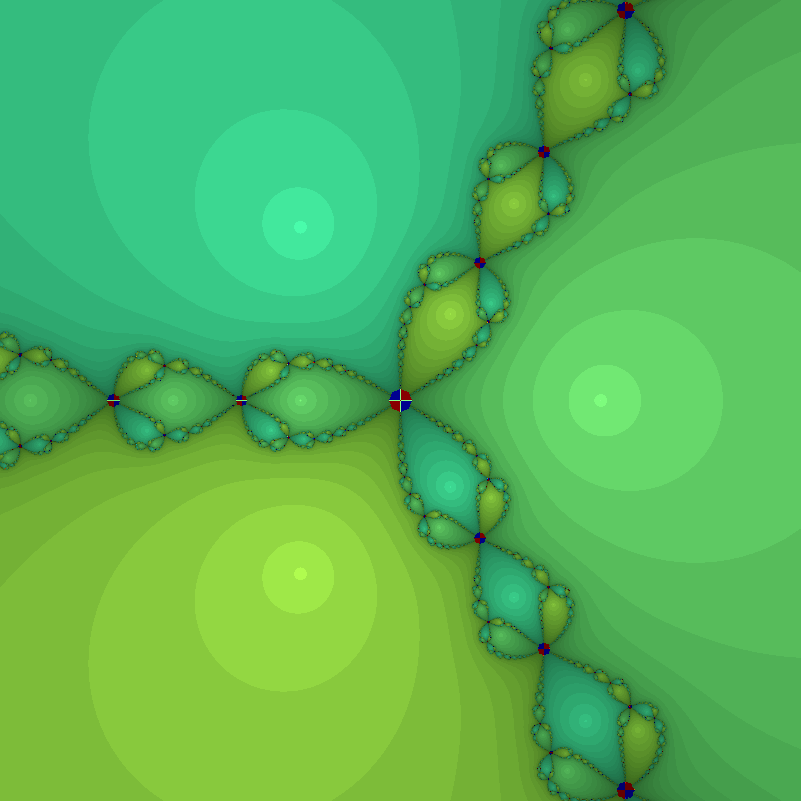Newton fractal for F=[x^3-3xy^2-1, 3x^2y-y^3]. Red and blue circles mark regions where iterates venture far from the origin.It is interesting to see how much perturbing it causes a modernist shift. If we use$F=[x^3-3xy^2-1 + \epsilon x, 3x^2y-y^3]$, then for$\epsilon=1$ we get: